SBOS709A July   2016  – July 2016

PRODUCTION DATA.

1. Features
2. Applications
3. Description
4. Revision History
5. Device Comparison Table
6. Pin Configuration and Functions
7. Specifications
8. Parameter Measurement Information
9. Detailed Description
1. 9.1 Overview
2. 9.2 Functional Block Diagram
3. 9.3 Feature Description
4. 9.4 Device Functional Modes
5. 9.5 Programming
6. 9.6 Register Maps
10. 10Application and Implementation
1. 10.1 Application Information
2. 10.2 Typical Applications
1. 10.2.1 DOCSIS 3.X Driver
3. 10.3 Do's and Don'ts
11. 11Power Supply Recommendations
12. 12Layout
13. 13Device and Documentation Support
14. 14Mechanical, Packaging, and Orderable Information

• RHA|40
• RHA|40

## 7 Specifications

### 7.1 Absolute Maximum Ratings

over operating free-air temperature range (unless otherwise noted)(1)
MIN MAX UNIT
Power supply –0.5 5.5 V
Input applied to analog inputs INPA, INMA, INPB, INMB –0.5 5.5 V
Voltage applied to input pins –0.5 5.5 V
Digital input/output voltage range –0.3 2 V
Operating junction temperature, TJ 125 °C
Storage temperature, Tstg –40 125 °C
(1) Stresses beyond those listed under Absolute Maximum Ratings may cause permanent damage to the device. These are stress ratings only, which do not imply functional operation of the device at these or any other conditions beyond those indicated under Recommended Operating Conditions. Exposure to absolute-maximum-rated conditions for extended periods may affect device reliability.

### 7.2 ESD Ratings

VALUE UNIT
V(ESD) Electrostatic discharge Human body model (HBM), per ANSI/ESDA/JEDEC JS-001(1) ±2000 V
Charged device model (CDM), per JEDEC specification JESD22-C101(2) ±1000
(1) JEDEC document JEP155 states that 500-V HBM allows safe manufacturing with a standard ESD control process.
(2) JEDEC document JEP157 states that 250-V CDM allows safe manufacturing with a standard ESD control process.

### 7.3 Recommended Operating Conditions

over operating free-air temperature range (unless otherwise noted)
MIN NOM MAX UNIT
VS Power-supply voltage 4.75 5 5.25 V
Specified operating temperature range –40 85 °C

### 7.4 Thermal Information

THERMAL METRIC(1) LMH2832 UNIT
RHA (VQFN)
40 PINS
RθJA Junction-to-ambient thermal resistance 29.5 °C/W
RθJC(top) Junction-to-case (top) thermal resistance 20.4 °C/W
RθJB Junction-to-board thermal resistance 6.6 °C/W
ψJT Junction-to-top characterization parameter 0.3 °C/W
ψJB Junction-to-board characterization parameter 6.5 °C/W
RθJC(bot) Junction-to-case (bottom) thermal resistance 2.3 °C/W

### 7.5 Electrical Characteristics

at TA = 25°C, VS+ = 5 V, Ri = 75 Ω, RL = 150 Ω, maximum gain (1:2-Ω ratio transformer plus 30-dB DVGA gain), f = 5 MHz to 300 MHz, VO converted to single-ended (SE) measurement with a transformer, and default current setting (unless otherwise noted); upon power-up, gain is set to mid-range
PARAMETER TEST CONDITIONS MIN TYP MAX UNIT TEST LEVEL(4)
AC PERFORMANCE
LSBW Large-signal,
–3-dB bandwidth
G = 30 dB, VO = 2 VPP 1140 MHz C
G = 0 dB, VO = 2 VPP 2350 C
BW Bandwidth for 0.1-dB flatness 300 MHz C
HD2 Second-order harmonic distortion f = 100 MHz, VO = 2 VPP –88 dBc C
f = 200 MHz, VO = 2 VPP –76 C
f = 300 MHz, VO = 2 VPP –63 C
f = 450 MHz, VO = 2 VPP –58 C
HD3 Third-order harmonic distortion f = 100 MHz, VO = 2 VPP –94 dBc C
f = 200 MHz, VO = 2 VPP –90 C
f = 300 MHz, VO = 2 VPP –81 C
f = 450 MHz, VO = 2 VPP –75 C
IMD3 Third-order intermodulation distortion f = 100 MHz, tone spacing = 2 MHz, POUT(1) = 0 dBm per tone –106 dBc C
f = 200 MHz, tone spacing = 2 MHz –102 C
f = 300 MHz, tone spacing = 2 MHz –86 C
OIP3 Output third-order intercept point f = 100 MHz, tone spacing = 2 MHz,
POUT = 0 dBm per tone
53 dBm C
f = 200 MHz, tone spacing = 2 MHz,
POUT = 0 dBm per tone
51 C
f = 300 MHz, tone spacing = 2 MHz,
POUT = 0 dBm per tone
43 C
P1dB 1-dB compression point f = 100 MHz, RL = 150 Ω 16 dBm C
f = 200 MHz, RL = 150 Ω 16 C
f = 300 MHz, RL = 150 Ω 16.5 C
NF Noise figure Ri = 150 Ω, f = 300 MHz, max gain 6.5 dB C
Output-referred voltage noise f = 300 MHz, max gain 47.7 nV/√Hz C
S11 Input return loss f = 300 MHz 17 dB C
S22 Reverse Isolation Including input transformer, f < 300 MHz 53 dB C
Channel-to-channel crosstalk f = 300 MHz, channel A to B –77 dB C
f = 300 MHz, channel B to A –81
Channel-to-channel phase matching f = 200 MHz ±0.1 ° C
Channel-to-channel gain matching f = 200 MHz ±0.05 dB C
GAIN PARAMETERS
Maximum voltage gain f = dc, gain code = 00h 29.5 30 30.5 dB A
Minimum voltage gain f = dc, gain code = 27h –9.5 –9 –8.5 dB A
Gain range 39 dB C
Gain step size Between any two adjacent gain settings 0.75 1 1.25 dB A
EG Gain error For any gain value –0.5 0 0.5 dB A
Cumulative gain error Referenced to max gain –1 1 dB A
Gain step transition time 6 ns C
zin Input resistance f = dc, differential 135 150 165 Ω A
Cin Input capacitance Differential 0.6 pF C
Single-ended input resistance f = dc 67.5 75 82.5 Ω A
Single-ended input capacitance 1.2 pF C
VICM Input common-mode voltage Internally biased to mid-supply –0.2 0.2 V A
VIL Low-level input voltage range Differential gain shift < 1 dB (VS–) + 1.5 V C
VIH High-level input voltage range Differential gain shift < 1 dB (VS+) – 1.5 V C
ANALOG OUTPUT CHARACTERISTICS
zo Output resistance Differential 20 Ω C
VOL Low-level output voltage range VS = 5 V, GND = 0 V 1.15 1.25 V A
VOH High-level output voltage range VS = 5 V, GND = 0 V 3.75 3.85 V A
VOM Maximum output voltage swing TA = 25°C 5 5.4 V A
TA = –40°C to +85°C 5.4 6.4 B
CMRR Common-mode rejection ratio 56 dB C
POWER SUPPLY
VS Supply voltage 4.75 5.0 5.25 V A
IQ Quiescent current per channel Default current, default bias setting 102 105 108 mA A
Min current, lowest power setting 90 C
Max current, highest bias setting 108 C
±PSRR Power-supply rejection ratio(2) Gain = 30 dB –48 dB C
POWER-DOWN(3)
Power-down quiescent current (per channel) TA = 25°C 2.5 6 mA A
TA = –40°C to +85°C 6.5 B
Power-down bias current –2 –1 µA A
Turn-on time delay Time to VO = 90% of final value,
gain = 0 dB, VI = 2 V
55 ns C
Turn-off time delay Time to VO = 10% of original value,
gain = 0 dB, VI = 2 V
110 ns C
Forward isolation in PD mode f = 300 MHz –67 dB C
DIGITAL INPUTS/OUTPUTS
VIH High-level input voltage 1.4 2 V A
VIL Low-level input voltage –0.3 0.8 V A
VOH High-level output voltage IOH = –100 µA 1.65 V A
IOH = –2 mA 1.55 A
VOL Low-level output voltage IOL = 100 µA 0.1 V A
IOL = 2 mA 0.2 A
(1) POUT is the signal tone power at the output of the device.
(2) PSRR is defined with respect to a differential output.
(3) The device power-down function can be controlled by the PDx pins or by the power-down register accessible from the SPI interface.
(4) Test levels: (A) 100% tested at 25°C. Overtemperature limits by characterization and simulation. (B) Limits set by characterization and simulation. (C) Typical value only for information.

### 7.6 Timing Requirements: SPI

MIN TYP MAX UNIT
fS_C SCLK frequency(1) 0 25 50 MHz
tPH SCLK pulse duration, high 10 ns
tPL SCLK pulse duration, low 10 ns
tSU SDI setup 3 ns
tH SDO hold 3 ns
tIZ SDO tri-state 3 ns
tODZ SDO driven to tri-state(2) 0 10 20 ns
tOZD SDO tri-state to driven 0 2 5 ns
tOD SDO output delay(2) 0 10 12 ns
tCSS CS setup(3) 3 5 ns
tCSH CS hold 3 ns
tIAG Inter-access gap 20 ns
(1) Tested on the automated test equipment (ATE) only up to 25 MHz.
(2) Referenced to the negative edge of SCLK.
(3) Referenced to the positive edge of SCLK.

### 7.7 Typical Characteristics

at TA = 25°C, VS+ = 5 V, Ri = 75 Ω, RL = 150 Ω, maximum gain (1:2-Ω ratio transformer plus 30-dB DVGA gain), f = 5 MHz to 300 MHz, VO converted to single-ended (SE) measurement with transformer, and default current setting (unless otherwise noted); upon power-up, gain is set to mid-range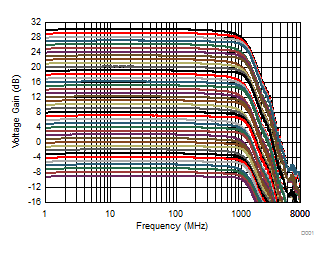VO = 2 VPP
Figure 1. Voltage Gain vs Frequency (1-dB Gain Steps)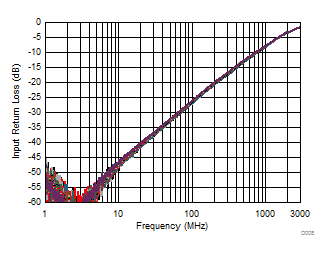Differential input impedance (ZIN) = 150 Ω, all gain settings
Figure 3. Input Return Loss vs Frequency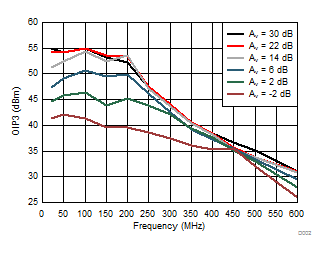POUT = 0 dBm per tone
Figure 5. OIP3 vs Frequency and Voltage Gain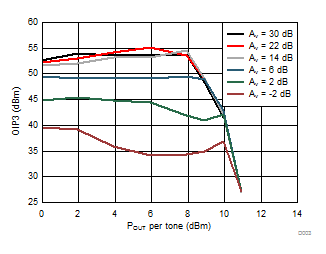f = 200 MHz
Figure 7. OIP3 vs Output Power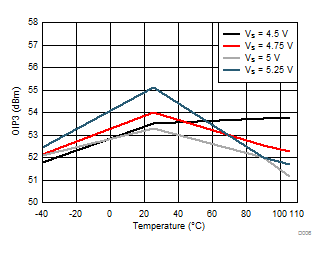POUT = 0 dBm per tone
Figure 9. OIP3 vs Temperature and Supply Voltage
(f = 200 MHz)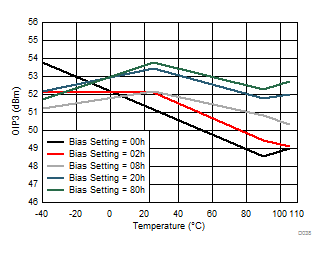POUT = 0 dBm per tone
Figure 11. OIP3 vs Temperature and Bias Setting
(f = 200 MHz)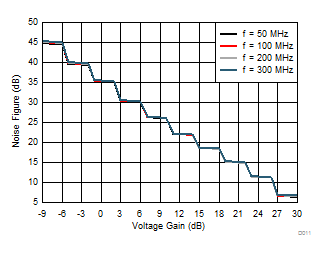ZIN = 150 Ω
Figure 13. Noise Figure vs Voltage Gain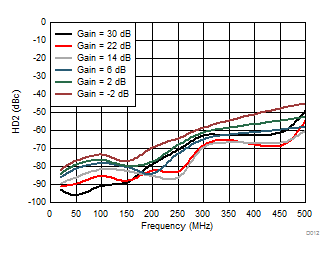VO = 2 VPP
Figure 15. HD2 vs Frequency and Gain Settings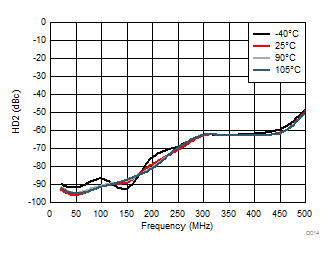VO = 2 VPP
HD2 vs Frequency and Temperature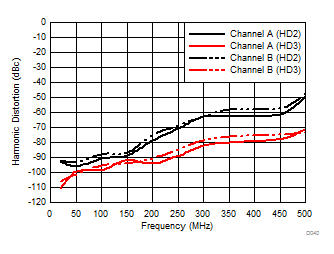Figure 18. Harmonic Distortion Between Channels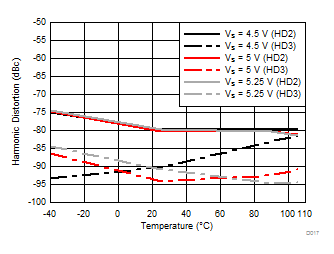VO = 2 VPP
Figure 20. Harmonic Distortion vs Temperature and
Supply Voltage (200 MHz)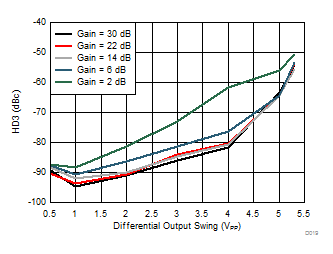Figure 22. HD3 vs Differential Output Swing (100 MHz)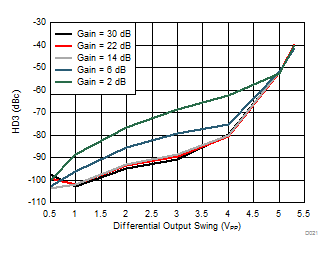Figure 24. HD3 vs Differential Output Swing (200 MHz)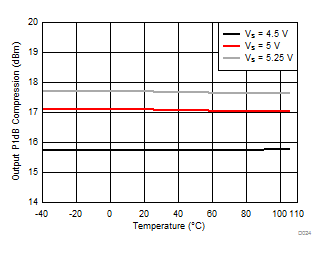Figure 26. Output P1dB Compression vs Temperature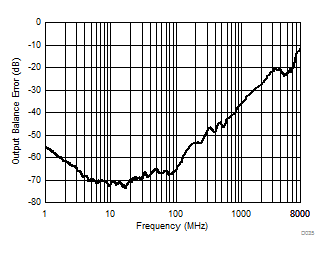Scd21 / Sdd21
Figure 28. Output Balance Error vs Frequency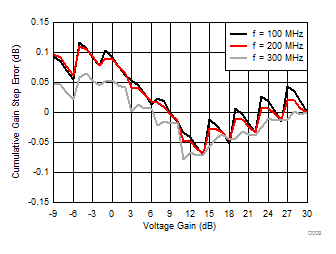Figure 30. Cumulative Gain Step Error vs Voltage Gain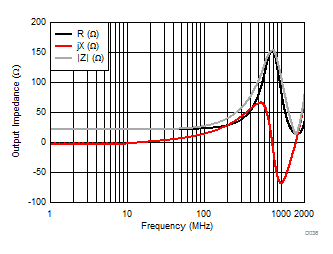Figure 32. Output Impedance vs Frequency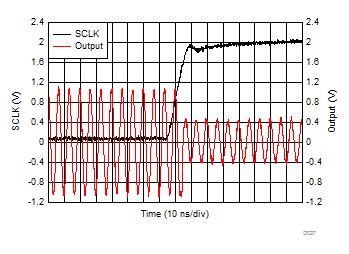Figure 34. Gain Switching Response (AV = 30 dB to 22 dB)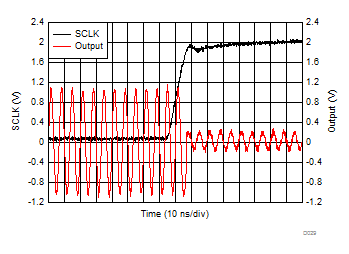Figure 36. Gain Switching Response (AV = 30 dB to 14 dB)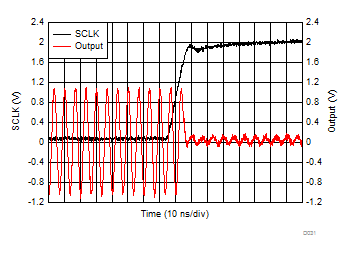Figure 38. Gain Switching Response (AV = 30 dB to 6 dB)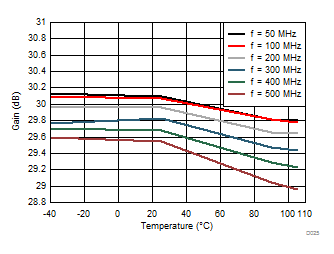Figure 2. Gain Flatness vs Temperature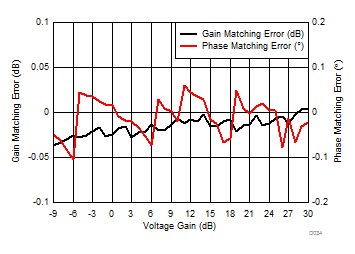f = 200 MHz
Figure 4. Channel-to-Channel Mismatch Error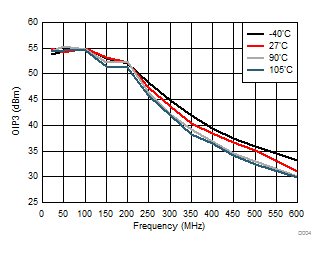POUT = 0 dBm per tone
Figure 6. OIP3 vs Frequency and Temperature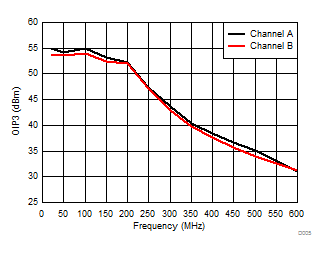POUT = 0 dBm per tone
Figure 8. OIP3 Channel Comparison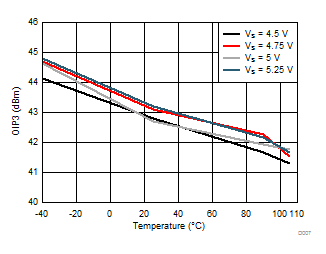POUT = 0 dBm per tone
Figure 10. OIP3 vs Temperature and Supply Voltage
(f = 300 MHz)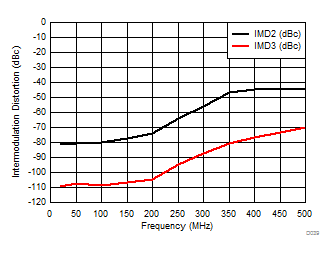POUT = 0 dBm per tone
Figure 12. Intermodulation Distortion vs Frequency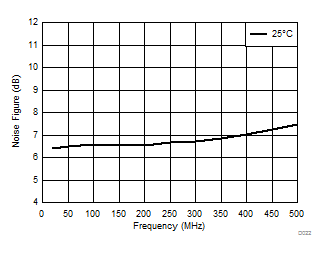Figure 14. Noise Figure vs Frequency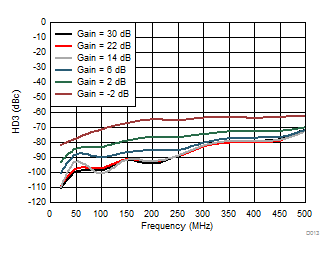VO = 2 VPP
Figure 16. HD3 vs Frequency and Gain Settings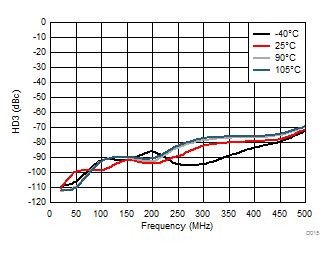VO = 2 VPP
Figure 17. HD3 vs Frequency and Temperature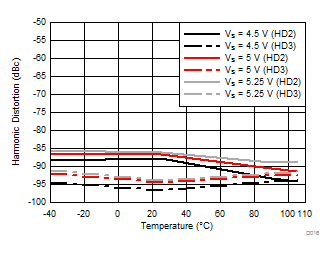VO = 2 VPP
Figure 19. Harmonic Distortion vs Temperature and
Supply Voltage (100 MHz)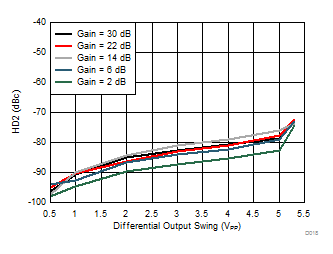Figure 21. HD2 vs Differential Output Swing (100 MHz)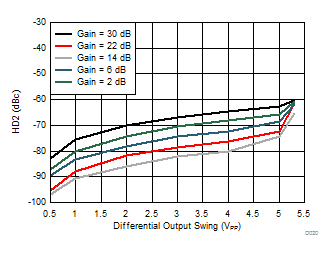Figure 23. HD2 vs Differential Output Swing (200 MHz)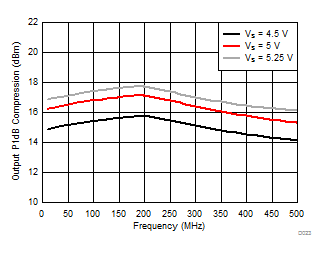Figure 25. Output P1dB Compression vs Frequency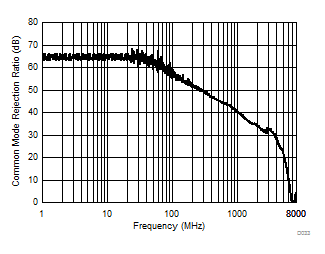Sdd21 / Scc21
Figure 27. CMRR vs Frequency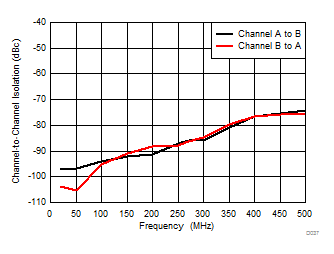Figure 29. Channel-to-Channel Isolation vs Frequency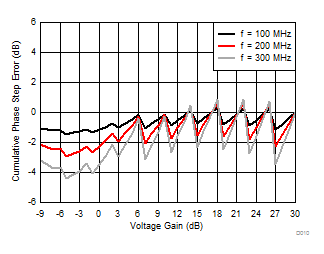Figure 31. Cumulative Phase Step Error vs Voltage Gain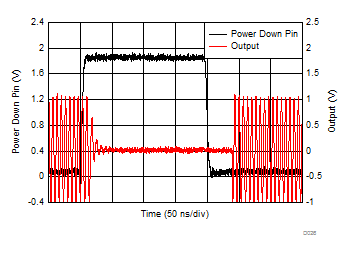Figure 33. Power-Down Transition ResponseFigure 35. Gain Switching Response (AV = 22 dB to 30 dB)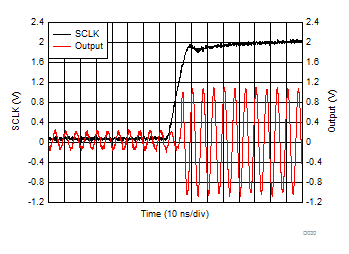Figure 37. Gain Switching Response (AV = 14 dB to 30 dB)Figure 39. Gain Switching Response (AV = 6 dB to 30 dB)